## Efficiency for Reijo's observation pattern sims

### 2019 May 1 - Clem Pryke

In these two postings Reijo posted results from observation pattern simulations "Pole wide" and "Chile narrow".

From these one can extract $$f_\mathrm{sky}$$ numbers for noise and signal and use these in Fisher calculations of $$\sigma(r)$$. In such calculations so far I think these hit patterns are being treated simply as "relative hits". However, we can get more from them than that:

• The sims are for one year of wall clock time dropping turnarounds and periods when no observation was scheduled.
• The sims involve observing at a range of elevation angles - and thus through varying air-mass. Lower elevation results in increased noise. As well as the actual hit time per pixel a variance map is also reported using NET which varies with elevation angle according to the 95GHz curves shown in this posting.

We can back out to the effective observing time per pixel using: \begin{equation} t_\mathrm{eff}=\frac{N^2_\mathrm{el=56}}{V} \end{equation}

where $$N_\mathrm{el=56}$$ is the NET at 56 degrees elevation (a nominal value whish is the average for BICEP/Keck), and $$V$$ is the reported Variance map. If the telescope spends all its time at lower elevations we will get a number less than one, and if it spends time higher we can get a number (modestly) greater than one. Here are the plots for "Pole wide" - the wall clock fraction is 88% and once the elevation penalty is added we get 82%. The ratio map comes out to exactly unity at Dec=-56 degrees as it should since at Pole the mapping bewteen Dec and elevation is simple.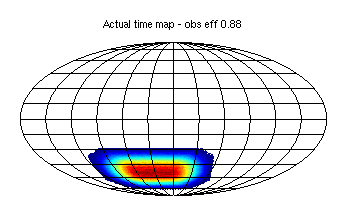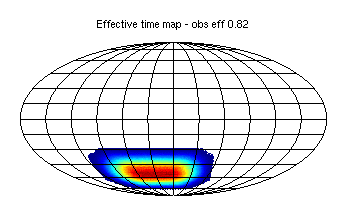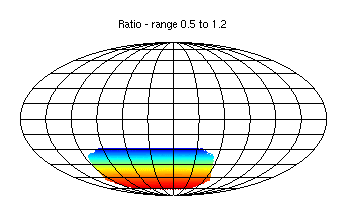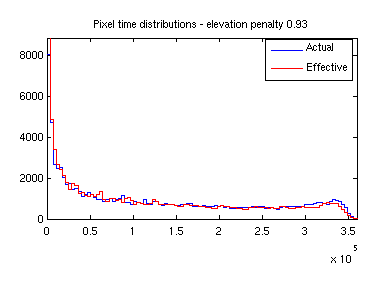Here are the plots for "Chile narrow" on the same color scales. Apparently the absolute observing efficiency is lower at 72% (I guess the telescope spends some time doing nothing? Reijo should explain). The penalty for hitting lower elevations is actually a little better and the overall efficiency is 69%.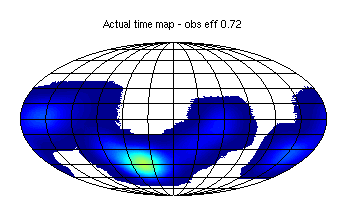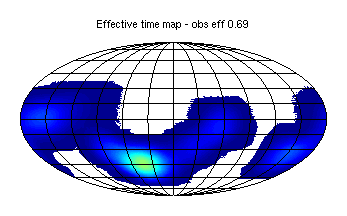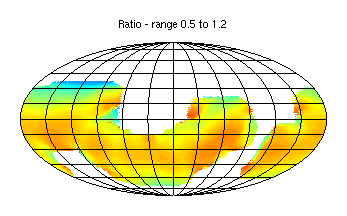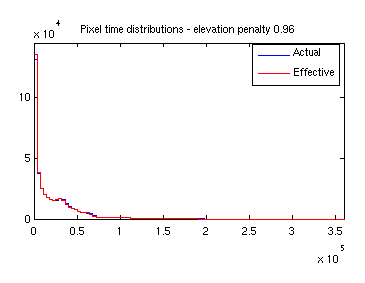### How to use the above?

For making map based sims scaling from BK15 achieved performance here is what we have been doing:

1. Take the actual BK15 reciprocal variance map and scale it to unit peak value - call this $$m$$.
2. Take the actual BK15 noise spectra and scale them by $$\sqrt{\sum{m^2}/\sum{m}}$$.
3. Generate full sky map realizations using these spectra.
4. Divide these by $$\sqrt{m}$$ to blow up the noise in the lower observation regions.

The sequence of above steps means that when one re-analyzes the maps using $$m$$ as the weights the BK15 noise spectra will be recovered exactly. This is what was done in this posting.

To change to a different amount of observing we scale the noise spectra by the ratio of detector-years versus BK15 before step 2 and repeat the subsequent steps .

To vary the sky coverage we can prepare alternate hit patterns with any distribution we want but normalized to the same sum as $$m$$ - no re-normalization to unit peak. This ensures exactly the same amount of assumed observing time is re-deployed on the sky. This is what was done in this posting.

I think to include the additional efficiency factors identified above one should start with the "Actual time maps" above, and, after normalizing them to the same sum as BK15 $$m$$, apply additional small factors:

1. The process above already includes the time inefficency of BK15 (at Pole) including turn-arounds etc etc. So presumably the Chile pattern should be adjusted to sum to 0.72/0.88=0.82 times the sum of $$m$$ to account for the time when no observation was scheduled. This is what would have happened if Reijo had instead hit some other (non-useful) part of the sky.
2. The Pole and Chile patterns should then be multipled by the elevation inefficiency ratio maps above. This is the correct thing to do since a deep region should not suffer a penalty just because some edge region is mostly observed at low elevation.

One might worry that the elevation penalty of BK15 which is already built into the BK15 noise spectra is getting double counted in step 2 above. But I think it is actually OK since we have deliberately used the mean elevation of BK15 (56 degrees) as the fiducial elevation, and the elevation range of BK15 is small (see plot here).

How to apply these factors to the Fisher type calcs is not completely clear to me right now. The Chile "no observation scheduled" time needs to get added to the "Actual time map" somehow - could be by just adding it in to the low observing region. This will slightly increase the $$f_\mathrm{sky}$$ numbers I guess. For the elevation penalty I'm not sure what to do - it doesn't just change the geometrical shape of the hit map - it actually increases/decreases the effective number of detector-seconds of observation in specific regions of the map. However, for Chile it is clearly a small effect in the deep region for the observation pattern considered above.